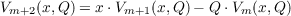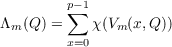#### Vol. 25, No. 3, 1968

 Download this articleFor screen For printingRecent Issues Vol. 325: 1 Vol. 324: 1  2 Vol. 323: 1  2 Vol. 322: 1  2 Vol. 321: 1  2 Vol. 320: 1  2 Vol. 319: 1  2 Vol. 318: 1  2Online Archive Volume: Issue:The Journal Subscriptions Editorial Board Officers Contacts Submission Guidelines Submission Form Policies for Authors ISSN: 1945-5844 (e-only) ISSN: 0030-8730 (print) Special Issues Author Index To Appear Other MSP Journals
Theorems on Brewer sums

### Stanley F. Robinson

Vol. 25 (1968), No. 3, 587–596
##### Abstract

Let V m(x,Q) be the polynomial determined by the recurrence relation(1)

(m = 1,2,), Q an integer, with V 1(x,Q) = x and V 2(x,Q) = x2 2Q. In a recent paper, B. W. Brewer has defined the sum(2)

where χ(s) denotes the Legendre symbol (s∕p) with p and odd prime.

The purpose of this paper is to consider the evaluation of Λ2n(Q) when n is odd. The principle result obtained is the expression of Λ2n(Q) as the sum of χ(Q) Λn(1) and one half the character sum ψ2e(1). ψ2e(1) can in turn be expressed in terms of the Gaussian cyclotomic numbers (i,j). The values of Λ6(Q) and Λ10(Q) follow immediately from this result utilizing values for Λ3(1) = Λ3 and Λ5(1) = Λ5 computed by B. W. Brewer and A. L. Whiteman.

Primary: 10.41
##### Milestones